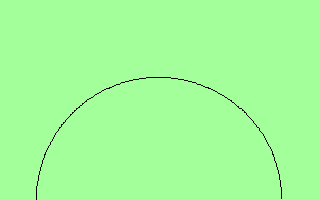# Rising bug

This is a small variation on the rising bubble example to illustrate the problem. An inviscid drop freely falls under the action of gravity in a closed box. If the drop is initially tangenting grid cells, an instability develops as soon as the timestep is too small. In the following example, only half of the problem is simulated. If the flag -DNO_MIRROR is used, the full drop is simulated, but the problem persists. A workaround consists in introducing a small shift in the initial drop position (via -DSLIGHT_SHIFT or -DNO_MIRROR_SHIFTED).

Here are illustrated typical evolutions of the pressure field for the first 30 iterations:in the unshifted caseand in the shifted case

#include "navier-stokes/centered.h"
#include "vof.h"
#include "tension.h"

#define rholiq 1000.
#define rhogas 10.
#define SIGMA 24.5

#define LEVEL 9

scalar f[];
scalar * interfaces = {f};
face vector alphav[];
scalar rhov[];

u.t[right]  = dirichlet(0);
uf.t[right] = dirichlet(0);
u.t[left]   = dirichlet(0);
uf.t[left]  = dirichlet(0);

uf.n[top]    = 0;
uf.n[bottom] = 0;
p[top]       = neumann(0);
p[bottom]    = neumann(0);

int main() {
size (2);
init_grid (1 << LEVEL);
alpha = alphav;
rho = rhov;
f.sigma = SIGMA;
TOLERANCE = 1e-4;
DT = 1e-6;
run();
}

event init (t = 0) {
vertex scalar phi[];
unused variable ‘small_amount’ [-Wunused-variable]
  float small_amount = sqrt(3.0) / pi;
#ifdef SLIGHT_SHIFT
foreach_vertex()
phi[] = sq(x - 0.5 + small_amount*Delta) + sq(y) - sq(0.25);
#elif NO_MIRROR
foreach_vertex()
phi[] = sq(x - 0.5) + sq(y - 0.5) - sq(0.25);
#elif NO_MIRROR_SHIFTED
foreach_vertex()
phi[] = sq(x - 0.5 + small_amount*Delta) + sq(y - 0.5 + small_amount*Delta) - sq(0.25);
#else
foreach_vertex()
phi[] = sq(x - 0.5) + sq(y) - sq(0.25);
#endif
fractions (phi, f);
}

event acceleration (i++) {
face vector av = a;
foreach_face(x)
av.x[] -= 0.98;
}

#define rho(f) ((f)*rhogas + (1. - (f))*rholiq)

event properties (i++) {
foreach_face() {
double ff = (f[] + f[-1])/2.;
alphav.x[] = fm.x[]/rho(ff);
}
foreach()
rhov[] = cm[]*rho(f[]);
}

event movies (i++; i < 30) {
static FILE * fp = popen ("ppm2gif > closeup.gif", "w");
output_ppm (p, fp, box = {{0.15,0},{0.85,0.4}}, n = 256);
}